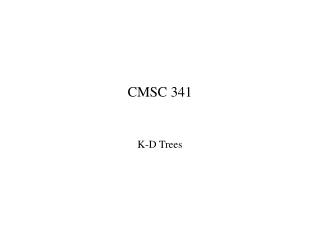DownloadDownload PresentationCMSC 341

# CMSC 341

Télécharger la présentation## CMSC 341

- - - - - - - - - - - - - - - - - - - - - - - - - - - E N D - - - - - - - - - - - - - - - - - - - - - - - - - - -
##### Presentation Transcript

1. CMSC 341 K-D Trees

2. K-D Tree • Introduction • Multiple dimensional data • Range queries in databases of multiple keys: Ex. find persons with 34 age 49 and \$100k annual income \$150k • GIS (geographic information system) • Computer graphics • Extending BST from one dimensional to k-dimensional • It is a binary tree • Organized by levels (root is at level 0, its children level 1, etc.) • Tree branching at level 0 according to the first key, at level 1 according to the second key, etc. • KdNode • Each node has a vector of keys, in addition to the two pointers to its left and right subtrees.

3. 99, 90 70, 3 53, 14 27, 28 65, 51 31, 85 30, 11 38, 23 29, 16 40, 26 7, 39 32, 29 82, 64 73, 75 15, 61 K-D Tree • A 2-D tree example

4. K-D Tree Operations • Insert • A 2-D item (vector of size 2 for the two keys) is inserted • New node is inserted as a leaf • Different keys are compared at different levels • Find/print with an orthogonal (square) range • exact match: insert (low[level] = high[level] for all levels) • partial match: (query ranges are given to only some of the k keys, other keys can be thought in range  ) high key key low high low

5. K-D Tree Insertion • template <class Comparable> • void KdTree <Comparable>::insert(const vector<Comparable> &x) • { • insert( x, root, 0); • } • template <class Comparable> • void KdTree <Comparable>:: • insert(const vector<Comparable> &x, KdNode * & t, int level) • { • if (t == NULL) • t = new KdNode(x); • else if (x[level] < t->data[level]) • insert(x, t->left, 1 – level); • else • insert(x, t->right, 1 – level); • }

6. 29, 16 7, 39 40, 26 32, 29 70, 3 38, 23 31, 85 73, 75 27, 28 15, 61 30, 11 Insert (55, 62) into the following 2-D tree 53, 14 65, 51 99, 90 82, 64 55,62

7. K-D Tree: printRange • /** • * Print items satisfying • * low <= x <= high and • * low <= x <= high • */ • template <class Comparable> • void KdTree <Comparable>:: • printRange(const vector<Comparable> &low, • const vector<Comparable> & high) const • { • printRange(low, high, root, 0); • }

8. K-D Tree: printRange (cont’d) • template <class Comparable> • void KdTree <Comparable>:: • printRange(const vector<Comparable> &low, • const vector<Comparable> &high, • KdNode * t, int level) • { • if (t != NULL) • { • if (low <= t->data && high >= t->data • && low <= t->data && high >= t->data) • cout << “(” << t->data ”,” • << t->data << “)” << endl; • if (low[level] <= t->data[level]) • printRange(low, high, t->left, 1 – level); • if (high[level] >= t->data[level]) • printRange(low, high, t->right, 1 – level); • } • }

9. 70, 3 53, 14 27, 28 29, 16 30, 11 73, 75 7, 39 82, 64 15, 61 99, 90 65, 51 printRange in a 2-D Tree In range? If so, print cell Low[level]<=data[level]->search t->left High[level] >= data[level]=> search t->right 31, 85 32, 29 40, 26 38, 23 low = 35, high = 40; This subtree is never searched low = 23, high = 30; Searching is “preorder”. Efficiency is obtained by “pruning” subtrees from the search.

10. K-D Tree Performance • Insert • Average and balanced trees: O(lg N) • Worst case: O(N) • Print/search with a square range query • Exact match: same as insert (low[level] = high[level] for all levels) • Range query: for M matches • Perfectly balanced tree: K-D trees: O(M + kN (1-1/k) ) 2-D trees: O(M + N) • Partial match in a random tree: O(M + N) where  = (-3 + 17) / 2

11. K-D Tree Performance • More on range query in a perfectly balanced 2-D tree: • Consider one boundary of the square (say, low) • Let T(N) be the number of nodes to be looked at with respect to low. For the current node, we may need to look at • One of the two children (e.g., node (27, 28), and • Two of the four grand children (e.g., nodes (30, 11) and (31, 85). • Write T(N) = 2 T(N/4) + c, where N/4 is the size of subtrees 2 levels down (we are dealing with a perfectly balanced tree here), and c = 3. • Solving this recurrence equation: T(N) = 2T(N/4) + c = 2(2T(N/16) + c) + c … = c(1 + 2 +  + 2^(log4 N) = 2^(1+ log4 N) – 1 = 2*2^(log4 N) – 1 = 2^ ((log2 N)/2) – 1 = O(N)

12. K-D Tree Remarks • Remove • No good remove algorithm beyond lazy deletion (mark the node as removed) • Balancing K-D Tree • No known strategy to guarantee a balanced 2-D tree • Tree rotation does not work here • Periodic re-balance • Extending 2-D tree algorithms to k-D • Cycle through the keys at each level